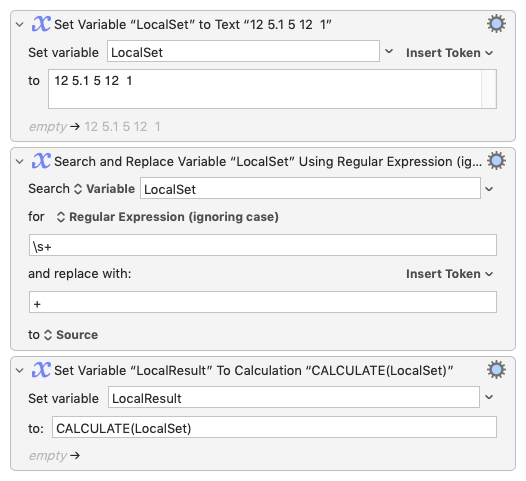# Calculating the number of number of numbers and calculating the sum

While I'm Okish at RegEx, I'm struggling with something that seems simple but is making my brain explode a touch

a set of numbers which could be up to 11 'groups' but more usually 5-6
for example

`12 5.1 5 12 2 1`

If I know there are 6 numbers I can `copy` the set of numbers, `Search System Clipboard Using Regular Expressions` to find

`([0-9.]+] ([0-9.]+] ([0-9.]+] ([0-9.]+] ([0-9.]+] ([0-9.]+]`

Setting each group as a variable `one, two, three, four, five, six`

Then I want the sum of those numbers to be calculated and pasted over them

`Insert Text %Calculate%one + two + three + four + five + six% by Pasting`

Which works fine.

But how can I calculate the number of numbers and turn them to variables? Or am I over complicating?

This is quite easy:

Replace the whitespace in your set of numbers with a `+` and then use KM to calculate the result like this:

Test Calculate.kmmacros (2.0 KB)The "pasting over them" I will leave as an exercise for you!

I forgot to say: I'm assuming all you really want to do is add up the numbers...

1 Like

Oh cool, will have a play, thank you!

In a scripting language you can typically use more `split` and less regular expression complexity:

Expand disclosure triangle to view JS Source
``````Application("Keyboard Maestro Engine")
.getvariable("numberString")
.split(/\s+/u)
.reduce((a, x) => a + parseFloat(x, 10), 0)
``````
1 Like

I would certainly never have got thereThank you both

1 Like

Is yours is really less complex?

My regex is `\s+` and stands alone
while yours is `/\s+/u` and is embedded in some JS.

No – addressing the OP : -)

Yes, of course. Sorry``````main :: IO ()